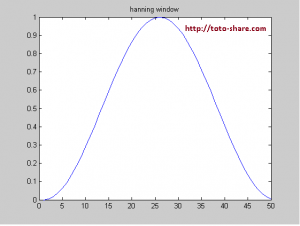# C/C++ : hanning Matlab Code

C/C++ : hanning Matlab Code

I have converted hanning.m code from Matlab to C/C++. The hanning.m is a function in Matlab created hanning window. This is my C/C++ hanning code converted from Matlab :

```/*  function w = hanning(varargin)
%   HANNING   Hanning window.
%   HANNING(N) returns the N-point symmetric Hanning window in a column
%   vector.  Note that the first and last zero-weighted window samples
%   are not included.
%
%   HANNING(N,'symmetric') returns the same result as HANNING(N).
%
%   HANNING(N,'periodic') returns the N-point periodic Hanning window,
%   and includes the first zero-weighted window sample.
%
%   NOTE: Use the HANN function to get a Hanning window which has the
%          first and last zero-weighted samples.ep
itype = 1 --> periodic
itype = 0 --> symmetric
default itype=0 (symmetric)

%   \$Revision: 1.11.4.3 \$  \$Date: 2007/12/14 15:05:04 \$
*/

float *hanning(int N, short itype)
{
int half, i, idx, n;
float *w;

w = (float*) calloc(N, sizeof(float));
memset(w, 0, N*sizeof(float));

if(itype==1)	//periodic function
n = N-1;
else
n = N;

if(n%2==0)
{
half = n/2;
for(i=0; i<half; i++) //CALC_HANNING   Calculates Hanning window samples.
w[i] = 0.5 * (1 - cos(2*PI*(i+1) / (n+1)));

idx = half-1;
for(i=half; i<n; i++) {
w[i] = w[idx];
idx--;
}
}
else
{
half = (n+1)/2;
for(i=0; i<half; i++) //CALC_HANNING   Calculates Hanning window samples.
w[i] = 0.5 * (1 - cos(2*PI*(i+1) / (n+1)));

idx = half-2;
for(i=half; i<n; i++) {
w[i] = w[idx];
idx--;
}
}

if(itype==1)	//periodic function
{
for(i=N-1; i>=1; i--)
w[i] = w[i-1];
w = 0.0;
}
return(w);
}```

This is the sample hanning windowing picture :You can download the complete C/C++ hanning code to create hanning window at here.